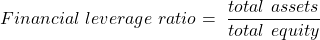Concept 48: Liquidity and Solvency Ratios | IFT World
101 Concepts for the Level I Exam

# Concept 48: Liquidity and Solvency RatiosLiquidity ratios measure a company’s ability to meet current liabilities. The higher the liquidity ratio, the more likely the firm will be able to meet its short term obligations.

• Current ratio – It is the most widely used measure of liquidity.

Current rati= current assets / current liabilities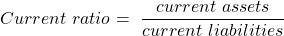• Quick ratio – It is a more conservative measure of liquidity. It excludes inventories and less liquid assets from the numerator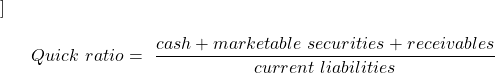• Cash ratio – It is the most conservative measure of liquidity. Even receivables are excluded from the numerator.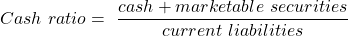Solvency ratios measure a company’s ability to meet long-term obligations. A high ratio indicates high leverage and a high financial risk.

• Long term debt to equity ratio — It measures long term financing sources relative to total equity.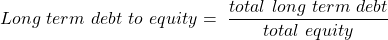• Debt to equity ratio– It measures total debt relative to total equity.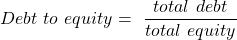• Total debt to assets ratio – It measures the extent to which assets are financed by liabilities.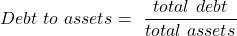• Financial leverage ratio – It measures total assets relative to total equity.